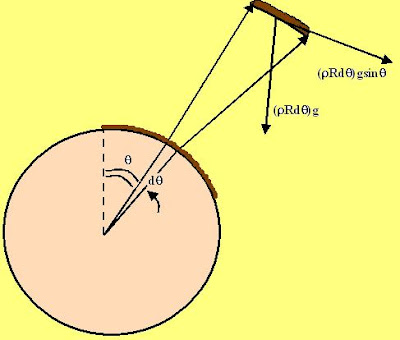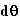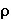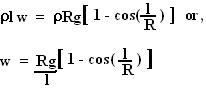## Monday, December 3, 2007

### Irodov Problem 1.107In this problem since there is no friction between the chain and the sphere, as soon as the chain is released, there is only one external force acting on the chain namely gravity and is solely responsible to accelerate the string. Basically we need to calculate the net force of gravity that is responsible in accelerating the string over the sphere. Since every part of the string experiences a different slope to slide on it experiences a different value of force responsible for sliding it along the sphere. The net force is thus the sum (integral) of forces acting on all the different part of the chain.

Consider an infinitesimally small part of the chain that subtends an anglefrom the center and is at an anglefrom the vertical as shown in the figure. The mass of this small piece of the chain is Thus the component of gravity responsible to slide this part of the chain over the sphere is, whereis the linear density (mass/unit length) of the chain assumed to be uniform all over the chain. This piece of chain feels as if it is placed on an inclined plane, inclined at an angleto the horizontal (you can use simple geometry to check this out). The component of gravity that is responsible for sliding this piece of the chain is given by. The net force on the chain is thus given by integrating this for every little piece in the chain. If the length of the chain is l, the angle it subtends is l/R and so the next force acting on the chain is given by,The total mass of the string is given by. So from Newton's laws we have,Here w is the acceleration of the chain.